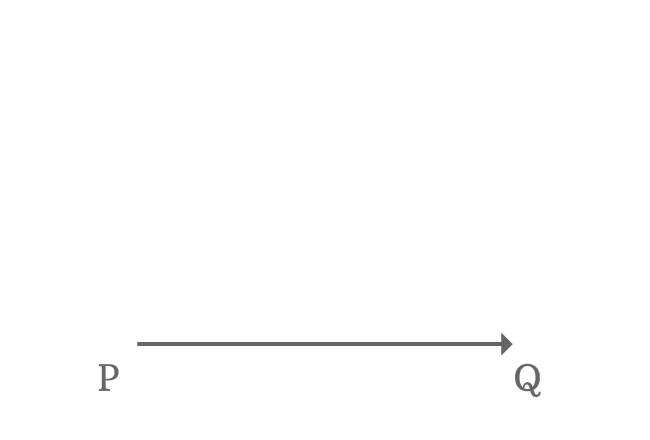# Angles

The amount of rotation of a ray from its initial position to final position is called angle.

###### Example$\overrightarrow{PQ}$ is a ray. It is rotated to another position from its initial position. Now, the ray $\overrightarrow{PQ}$ is called as $\overrightarrow{PR}$.

The amount of rotation of the ray $\overrightarrow{PQ}$ to reach its final position is called angle.

The graphical animation understood you the concept an angle in geometrical mathematics. Now, it is time to move you to the next level.

### Basics

Two terms are used to study the concept of an angle in geometrical mathematics.

### Representation

A special mathematical approach is used to represent an angle in mathematics.

### Formation

There are two different theories to understand the geometrical formation an angle.

### Sense

There are two kinds of angles and it is determined by the rotation of side or arm.

### Types of Angles

A complete rotation of a line is $360^\circ$. The angles are classified into seven types on the basis of the sectors and some notable angles of region of the complete rotation.

Latest Math Topics
Jun 26, 2023
Jun 23, 2023

Latest Math Problems
Jul 01, 2023
Jun 25, 2023
###### Math Questions

The math problems with solutions to learn how to solve a problem.

Learn solutions

Practice now

###### Math Videos

The math videos tutorials with visual graphics to learn every concept.

Watch now

###### Subscribe us

Get the latest math updates from the Math Doubts by subscribing us.